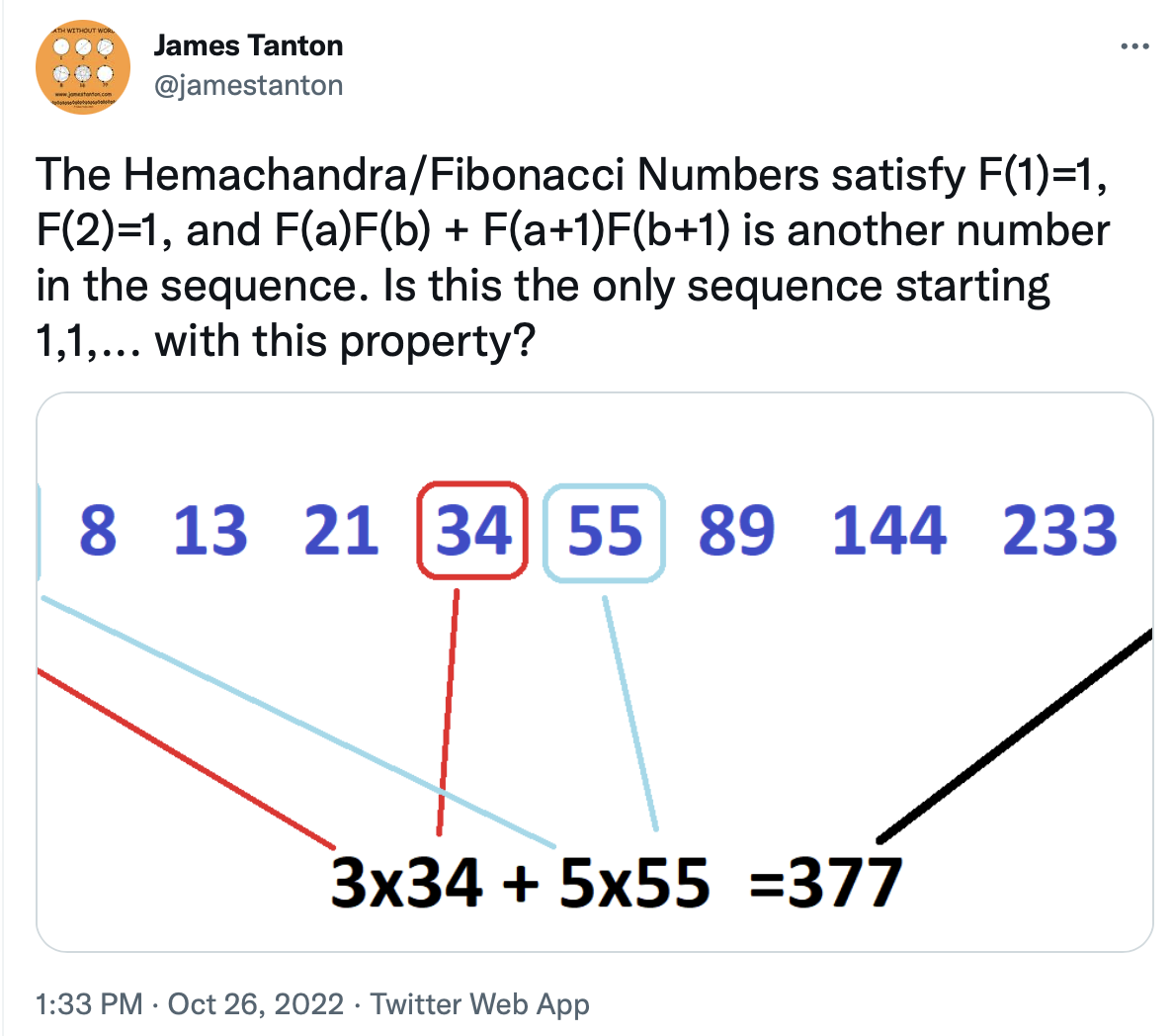# New Fibonacci-problem, product sum

James Tanton published a new problem about Fibonacci sequence  on Thursday. I started to play with it with GeoGebra. After I published my article in  Finnish some people asked about having it in English, so they could use it easier with their students. So here it is. Here I am working only with the traditional Fibonacci sequence from Liber Abaci.In Tanton’s example  a = 4, F(3) = 3, b = 9, F(9) = 34, F(5) = 5 ja F(10) = 55 ja 377 = F(14).

flista = {1, 1, 2, 3, 5, 8, 13, 21, 34, 55, 89, 144, 233, 377, …}

When I was playing with this with GeoGebra I noticed a simple connection how far a and b gets T(a,b) in the Fibonacci sequence. Kind of happy about that. Still no idea how to prove it.

At this point I will leave you some problems.

1. Tanton’s Theorem. Let F(n) be the n’th number in the traditional Fibonacci sequence and T(a, b) = F(a)*F(b) + F(a+1)*F(b+1), a>0, b>0 and of course they are natural numbers. Then T(a, b) is a Fibonacci number. So there exist a number n so that T(a, b) = F(n).
2. Find a relation between n, a and b, if the theorem is OK. In Tanton’s example a = 4 ja b = 9, T(4 , 9) = 377 = F(14).
3. Is thera a simple equation for T(a, b), without recursion.
4. Are there any other initial values F(1), F(2) for the sequence to have this property.

For me it is a little bit hard to find T(a,b) (problem 3). Tinkering (haha learnt a new word from Thomas’s book) with this idea I found that if a + b is constant, then T(a, b) is constant. So T(1,9) = T(2, 8) = T(3,7) = T (4,6) =T(5,5) = 89

I will guess that proving those theorems/problems are kind of hard.

I will be back.